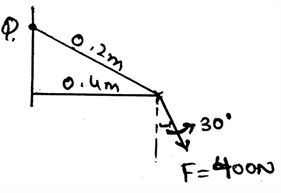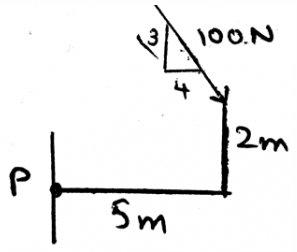Courses

# Test: Frictional Forces On Journal Bearings

## 15 Questions MCQ Test Engineering Mechanics | Test: Frictional Forces On Journal Bearings

Description
This mock test of Test: Frictional Forces On Journal Bearings for Mechanical Engineering helps you for every Mechanical Engineering entrance exam. This contains 15 Multiple Choice Questions for Mechanical Engineering Test: Frictional Forces On Journal Bearings (mcq) to study with solutions a complete question bank. The solved questions answers in this Test: Frictional Forces On Journal Bearings quiz give you a good mix of easy questions and tough questions. Mechanical Engineering students definitely take this Test: Frictional Forces On Journal Bearings exercise for a better result in the exam. You can find other Test: Frictional Forces On Journal Bearings extra questions, long questions & short questions for Mechanical Engineering on EduRev as well by searching above.
QUESTION: 1

### The journal bearings are generally used in __________

Solution:

The journal bearings are being used in the supports. They are used as to support the loadings. This means that the more the bearings the more is the stable the structure. Thus the use of journal bearings.

QUESTION: 2

### A phenomena is there in these collar bearings and pivots, which is very helpful in the supporting of the machines, and that is _____________

Solution:

The dry friction is acted upon the surfaces. And they are tangential to each other. As we know the friction is the phenomena that defines that there is a resistance which is present there between the two surfaces. This Coulomb friction is also known as dry friction.

QUESTION: 3

### Determine the moment about the point Q by the force shown as 400N.Solution:

As we know that the moment is the cross product of the force and the distance between the point of contact of the force and the point about which moment needs to be calculated. Thus forming the distance vector and then crossing it with the force will give us the answer. Remember force also needs to be in the vector form for doing the cross product.

QUESTION: 4

At what conditions does the Coulomb friction occurs between the surfaces in contact of journal bearing?

Solution:

When there is no lubricating fluid present between the surfaces in contact, the dry friction occurs. Thus as the name suggests, dry friction. When there is no liquid present over there the surfaces are going to be said dry only. Thus the term dry friction.

QUESTION: 5

We first make equilibrium equations of the sections involving the journal bearings by considering all the three dimensional forces acting on the section chosen and then the free body diagram is made and solved.

Solution:

We first make the free body diagram of journal bearings and then we make the equilibrium equations to satisfy the given conditions. This helps us to solve the question easily. As this reduces the part of imagination and increases accuracy too.

QUESTION: 6

To know the rotations involved in the journal bearings we use right handed coordinate system, in that system which of the following is followed (consider the mentioned axis to be positive)?

Solution:

As right handed coordinate system means that you are curling your fingers from positive x-axis towards y-axis and the thumb which is projected is pointed to the positive z-axis. Thus visualizing the same and knowing the basic members of axis will not create much problem.

QUESTION: 7

The moment is the cross product of which two vectors in the calculations of the journal bearings?

Solution:

The cross product needs to take in the proper sequence. If not taken then the answer is just the opposite of the true answer. That’s why, the answer is not the Force and Radius vectors, but the Radius and Force vectors. Because the moment has its direction, as many of the cross products have, and thus precaution needs to be taken.

QUESTION: 8

Determine the moment about the point P.Solution:

As we know that the moment is the cross product of the force and the distance between the point of contact of the force and the point about which moment needs to be calculated. Thus forming the distance vector and then crossing it with the force will give us the answer. Remember force also needs to be in the vector form for doing the cross product.

QUESTION: 9

The basic type of motion of a body is not the translation motion only.

Solution:

The basic types of the motion of the body are translation and rotational. In which translation means the motion is a straight motion while the in the rotational motion the body is moving of the body along the axis. The pivots do circular motion.

QUESTION: 10

Sometime the principle of transmissibility is somehow applied to journal bearings. It states that:

Solution:

Principle of transmissibility states that the force acting on the body is a sliding vector. That is it can be applied at any point of the body. It will give the same effect as if applied at any point other that the specific point. Thus the answer.

QUESTION: 11

Coplanar forces are not easily simplified in the simplification of the force and couple system in the calculations of forces in the journal bearings.

Solution:

The coplanar forces can be easily simplified in the simplification of the force and couple system. This is not only for the coplanar system of the forces, but for any of the dimension vector. Which means the answer is false.

QUESTION: 12

There are some of the applications of the two force and three force members in the journal bearings. The difference between the two and the three force members is:

Solution:

The definition of the two force member only defines that the forces are being acted on the two points on the body. So does is the definition of the three forces members. The points of action of the three forces are three.

QUESTION: 13

Which of the following is true for the determination of the rotational effect of the bearings?

Solution:

We can take such assumptions. We are free to do so. We just need to specify if clockwise is positive then the counter clockwise is to be negative. Also such assumption are very useful in 2D calculation of the moments caused by various forces on the body.

QUESTION: 14

The doors are having hinges to support them. This hinge is too a type of bearing or is a Device which is using effect like bearing to support. So, if you are opening the door, then the force you apply is greater if you open it from the part closer to the hinges.

Solution:

As the moment is the product of the force and the perpendicular distance of the point of action and the axis, thus more the distance more will be the moment. And hence to overcome that you have to apply more energy. Thus the answer.

QUESTION: 15

Which of the following is true for the bearing’s body?

Solution:

When we consider about the dimensions we need to be careful. The moment is the vector quantity. Thus the value of the total moment caused by various forces acting on the body is the vector sum of all the vectors. Also the moments are not perpendicular to each other, unless it is specified.